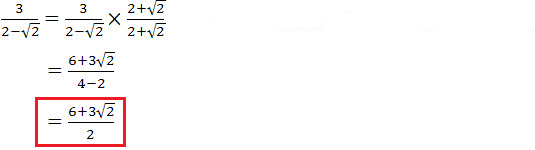# Engineering Mathematics Six Surds Rules pdf

An online surds rule printable

# What is Surds?

Surds are basically an expression involving a root, squared or cubed etc…There are some basic rules when dealing with surds

Example:  √36  =  6

The above roots have exact values and are called Rational

Example:  √2  =  1.41

These roots do NOT have exact values and are called Irrational OR SURDS

## Six Rules Of Surds:

 Rule 1: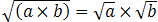Rule 2: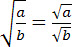Rule 3:Rule 4: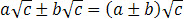Rule 5:Rule 6:## Example for 6 rules of Surds

Example For Rule 1:Simplify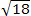:

Some square roots can be broken down into a mixture of integer values and surds. The following examples will illustrate this idea:Example For Rule 2:You may recall from your fraction work that the top line of a fraction is the numerator and the bottom line the denominator.

Simplify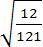:Note:If by using certain maths techniques we remove the surd from either the top or bottom of the fraction then we say we are “rationalising the numerator” or “rationalising the denominator”.

Remember the rule :√a  x  √a  =  √a

Example For Rule 3:Simplify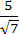:Example For Rule 4:Simplify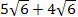:Example For Rule 5:Simplify: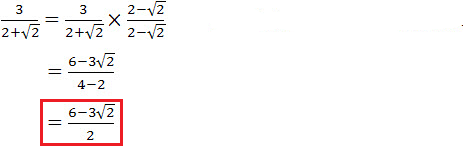Example For Rule 6:Simplify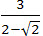: